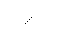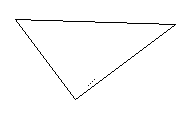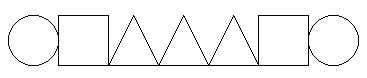# Structures in C

In this lab you will learn how to define and use C structures.

## Learning Objectives

By the end of this lab, you should be able to:

• Define a struct for encapsulating data.
• Create new types using enum.
• Use structs and enums to make your programs more readable.

## Starter code

The starter code for this lab can be found at the following links:

Download the starter code and unzip it. Make sure it compiles by running make. You will be editing the main.c file.

## Your first structure

You already know about primitive data types in C such as int, char, etc. You can create more complex data types using the struct keyword. A struct combines several pieces of data into a single aggregate data structure (thus the name).

struct point {
int x;
int y;
};

Question: What does this code remind you of from Java?

A struct allows us to abstract away some of the underlying memory layout details, collecting related information inside of a single structure. This is a form of encapsulation, a term you may remember from your Java courses.

Question: What is the benefit of encapsulation?

DO THIS: Fill in the code of the print_point function; it is a function that takes a parameter of type struct point, and prints out the x- and y-coordinates:

    void print_point(struct point p)
{
printf("Point: (%d, %d)\n", p.x, p.y);
}

This code can be tested using this following snippet:

    struct point the_point = {.x = 5, .y = 7};
print_point(the_point);

Try this yourself. Make sure that it prints what you expect and that you understand what each piece does.

## Key differences from Java

One key difference between a Java class and a C struct is that the struct cannot directly contain member methods. It can only contain member data. This is why C is not considered to be object-oriented: we cannot call methods on structs. This makes the language simpler but more limited.

Another major difference is that there is no notion of a "private" member variable. All variables are publicly-visible by default. It is possible to define a struct in a header file such that its members are not visible to files that include that header, but we will not be using such functionality in this lab.

## Initializing Structs

Note that initializing a struct can be done in multiple ways. It can be done inline, as was done above:

    struct point the_point = {.x = 5, .y = 7};

Here we create a new point with the x member set to 5 and the y member set to 7.

You can also create the struct without initialization and then modify its data afterwards, like:

    struct point the_point;
the_point.x = 5;
the_point.y = 7;

Question: What do you think happens if you do this:

    struct point the_point;
print_point(the_point);

## Accessing Attributes

Note that here the dot (.) syntax is used to access the struct's variables. These can also be used in computations. For instance, we could define a function that adds two points together:

     struct point add(struct point p1, struct point p2)
{
// Get the sums of the x and y values:
int sum_x = p1.x + p2.x;
int sum_y = p1.y + p2.y;

// Create a new point with the sum values:
struct point ret_point;
ret_point.x = sum_x;
ret_point.y = sum_y;

return ret_point;
}

Or, much more succinctly:

    struct point add(struct point p1, struct point p2)
{
struct point ret_point = {.x = p1.x + p2.x, .y = p1.y + p2.y};
return ret_point;
}

## Exercise: Drawing points revisited

Remember that in the last lab we used 2-dimensional arrays to create a list of points. This was a little cumbersome from a code-readability standpoint because when you look at a piece of code that reads "points" it is not really clear exactly what the indices are referring to.

Using structs, we can now make this a bit more readable. We can use our point type to create an array of points:

    struct point my_points = {
{.x = 13, .y = 14},
{.x = 15, .y = 16},
{.x = 17, .y = 18},
{.x = 19, .y = 20}
};

Now, rewrite your draw_points function from the last lab to use your new type struct point.

DO THIS: Fill in the code of the draw_points function:

    void draw_points(struct point points[], int num_points) {
}

The input to draw_points is an array (one dimensional) named points, containing num_points number of points.

As output, your function should draw all of the points in points using the turtle graphics library. You may use the turtle_draw_pixel function, or you may manually move the turtle using turtle_goto function and draw the point using the turtle_draw_dot function. See the link for documentation on these functions.

The resulting image should have four dots in a diagonal row, as below:Dots

### Exercise: distances between points

DO THIS: Fill in the code of the dist function:

    double dist(struct point p1, struct point p2);

This function takes as input two points (p1 and p2), calculating and returning the distance between them as a double.

Hint 1: Remember that the distance between two pointsandis given byHint 2: The math.h library file contains a function called sqrt that takes an integer (or a double) as a parameter and returns the square root of the number as a result. For instance sqrt(4) returns 2.0.

Check to make sure that the distance between the points in the testing code in main() is approximately 305.9.

## Typedefs for the win

Notice that every time we want to take a struct as a parameter to a function or define a struct we have to use the struct keyword. This starts to get cluttered. For instance, the signature for our add function above is:

    struct point add(struct point p1, struct point p2);

In Java, this would undoubtedly look a lot prettier, something like:

    Point add(Point p1, Point p2);

In such cases, the struct keyword is just cluttering things up. Fortunately C has a useful tool for dealing with this: the typedef. The typedef keyword allows you to define new types for your program.

For a silly example, you could do:

    typedef int integer;

That defines a new type called integer, which is basically just an alias for int. After adding that typedef, you could do this:

    integer x = 5;

More helpfully, programmers sometimes use typedef to create shorthand versions of types, for instance, rather than unsigned int you can use typedef unsigned int uint; to use uint as shorthand for unsigned int. This sort of shorthanding also works for for structs.

### Exercise: Create a point_t type using typedef.

DO THIS:

1. Create a new type called point_t using typedef as an alias for struct point.

2. Convert your code so that it no longer uses struct point but instead uses point_t as the type.

## Another way to create types: enum

Another useful method for creating types is with the enum keyword. The enum keyword allows you to create an enumerated type, or rather a type whose values come from a pre-defined finite list. You can also combine typedefs and enums.

Consider the following code:

    // Define a new type called fruit_t, which
// is either APPLES, ORANGES, or BANANAS:
//
typedef enum {
APPLES,
ORANGES,
BANANAS
} fruit_t;

void print_fruit(fruit_t which_fruit)
{
if (which_fruit == APPLES) {
printf("Apples!\n");
} else if (which_fruit == ORANGES) {
printf("Oranges!\n");
} else if (which_fruit == BANANAS) {
printf("Bananas!\n");
}
}

## Another (shorter) way to create typedef'ed structs

You may have noticed in the previous code example that we declare the enum and the typedef in one declaration, rather than two separate ones as we did with structs. The former syntax is cleaner and avoids any intermediate names for the enum. In fact, you can use it for structs as well. Here is our point_t type defined using this syntax:

    typedef struct {
int x;
int y;
} point_t;

### Drawing triangles

1. Create a struct called triangle_t for storing triangles. Each triangle should store three points: p1, p2, p3, which are its endpoints.

2. Write a function called draw_triangle, which takes as input a triangle_t and then draws the triangle using the turtle graphics library.

3. In your main() function, create a triangle with corners at (0,0), (100,75), (-60,80) using your new triangle_t type and draw it.

Your output should look like this:A triangle

### Icons

For this exercise you are going to create an icon_t type to define icons. An icon will have a shape (either CIRCLE, SQUARE, or TRIANGLE), a dimension (width x height). You will then write a draw_icons function that takes as input an array of of icon_ts and draws the icons along a row in the image.

1. Define a new enumerated type called shapetype_t which can be either CIRCLE, SQUARE, or TRIANGLE. (Hint: use enum.)

2. Define a new type called icon_t which stores a size_t called size and a shapetype_t called icon_type.

3. Define a function called draw_icons which takes as input an array of icons and the number of icons in the array and a starting (x, y) position and draws them in a row (according to their icon_type) using the turtle graphics library. Hint: the function's signature should be:

void draw_icons(icon_t icons[], int num_icons, int start_x, int start_y).

4. In your main() function add code to create an array called icons which has at least one icon of each type. Use your draw_icons function to draw the icons in the array.

Your output should look something like this:A row of seven icons